# Stata：Finance Topics

This articles is my notes for learning two three articles which introduce some technical analysis methods in Stata.

## Bollinger Bands

Bollinger bands were developed by John bollinger in the 1980s and consist of an upper and lower band, defining an envelope of usual trading. A stock price moving outside this envelope triggers an investment decision. The upper band is calculated as K standard deviations above the stock’s moving average. The lower band is calculated as K standard deviations below the moving average. bollinger bands mimic the idea that random variables tend to stay within plus or minus two standard deviations from their mean. To this insight, Bollinger bands add that the financial data might be not stationary, so the means and standard deviations are updated continually.

The usual trading system using Bollinger bands is to sell when the price reached it upper band, because a reversion to the mean is expected. A contrarian Bollinger trading system reverses this logic: buy when the price crosses its upper band.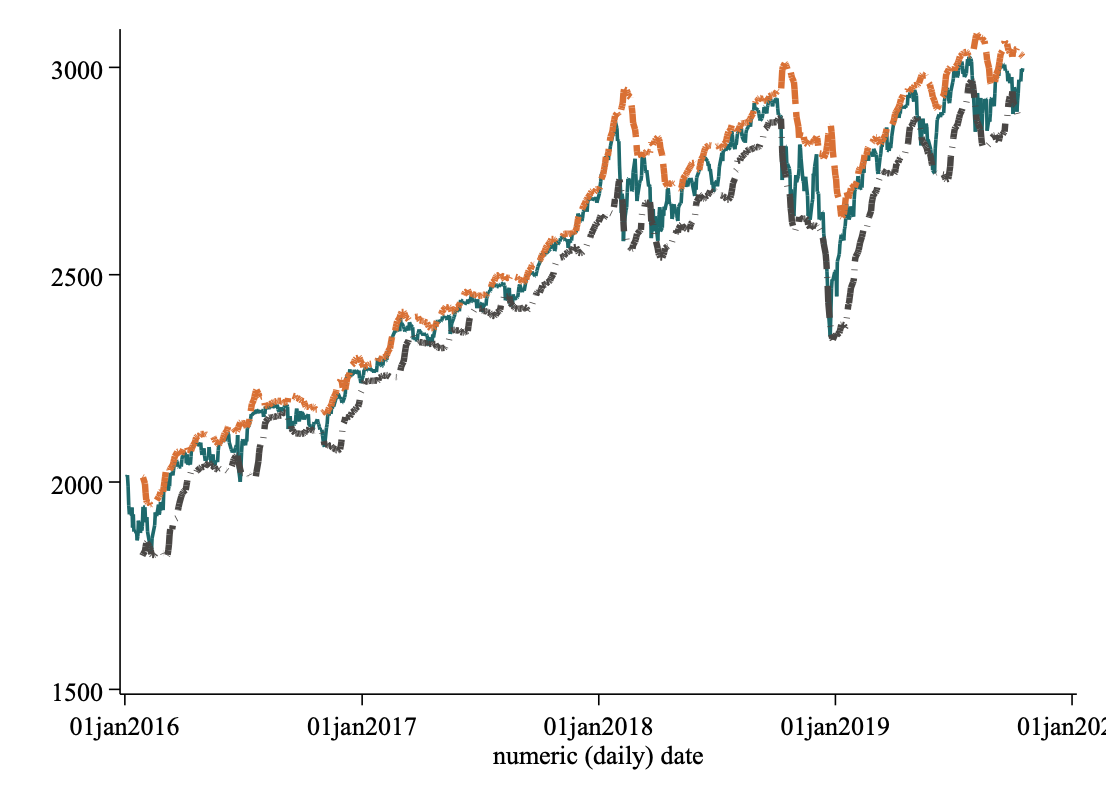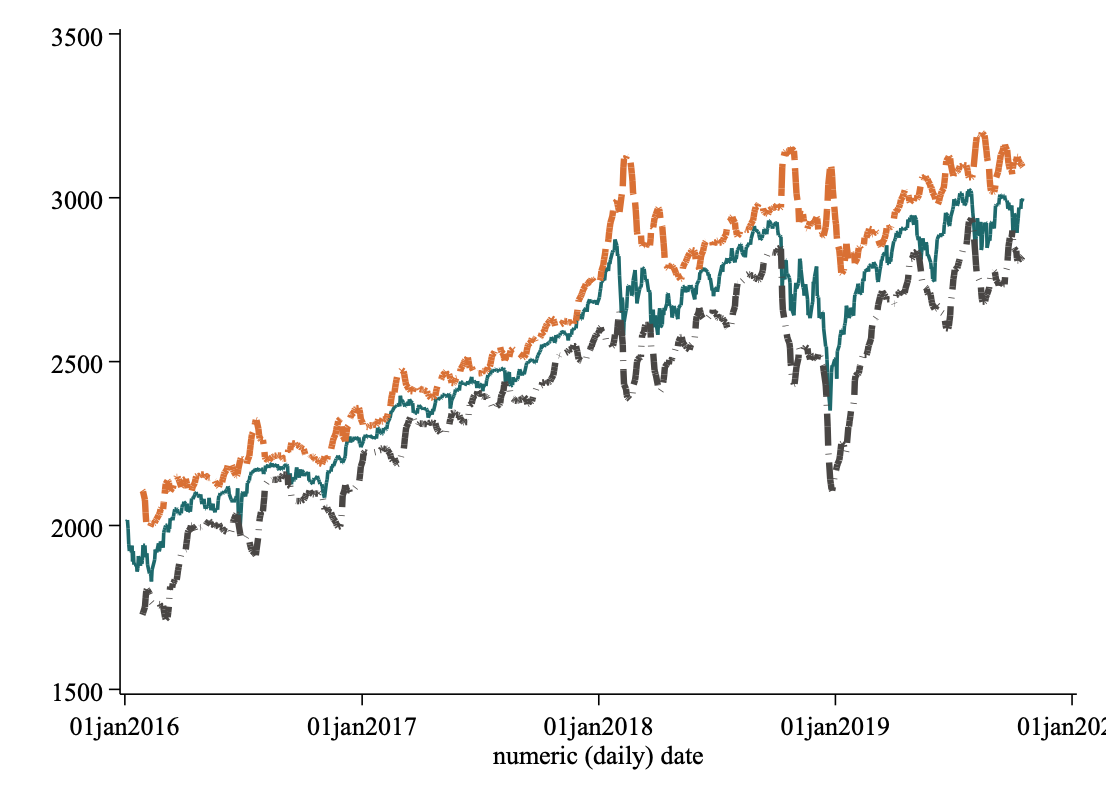## MACD

The MACD (“Mack-D”) is a momentum indicator commonly used by technical analysis. The MACD is equal to the difference between 2 EMAs of different lengths: (1) a long-term EMA of usually 26 days and (2) a short-term EMA of usually 12 days. The MACD is defined as the short-term EMA minus the long-term EMA. A third component is the so-called signal lines, which is usually a nine-day EMA of the MACD.

The convention is to plot the signal line over the MACD. A “bullish” indicator is when the signal line crosses above the MACD. A “bearish” indicator is when the signal line crosses below the MACD.

The MACD histogram is equal to the MACD less the signal line. As such, it oscillates above and below zero. A bullish signal is when the histogram becomes positive. A bearish signal is when the histogram becomes negative. The histogram is used as an indicator of momentum. When the histogram is positive ans increasing, the stock price is said to have increasing positive momentum. A negative and decreasing histogram implies steeper downside momentum.

It is often viewd as signaling the end of a current trend when the actual stock price diverges significantly from the MACD. Finally, the MACD rises steeply when the short-term EMA (usually 12 days) is rising faster than the long-term EMA (usually 26 days). That is, price have moved dramatically upward recently. Analysts sometimes infer that the current price has moved away from its equilibrium value, and they expect a downward correction.## Moving Average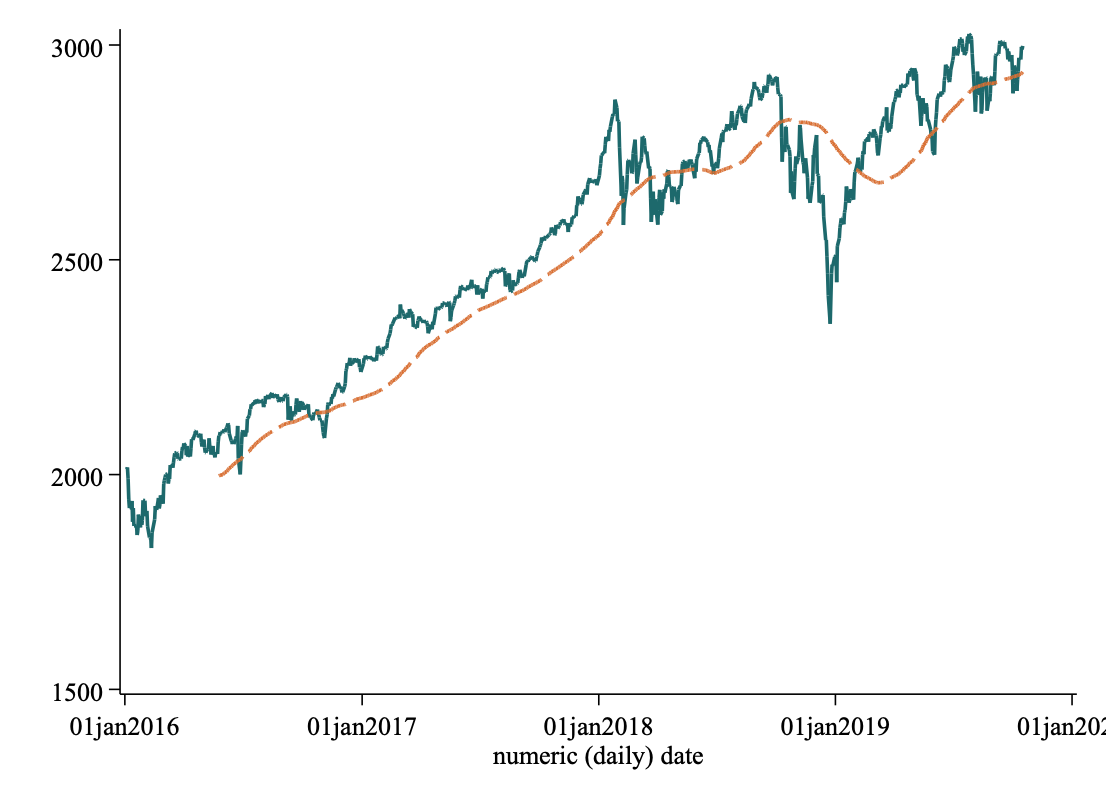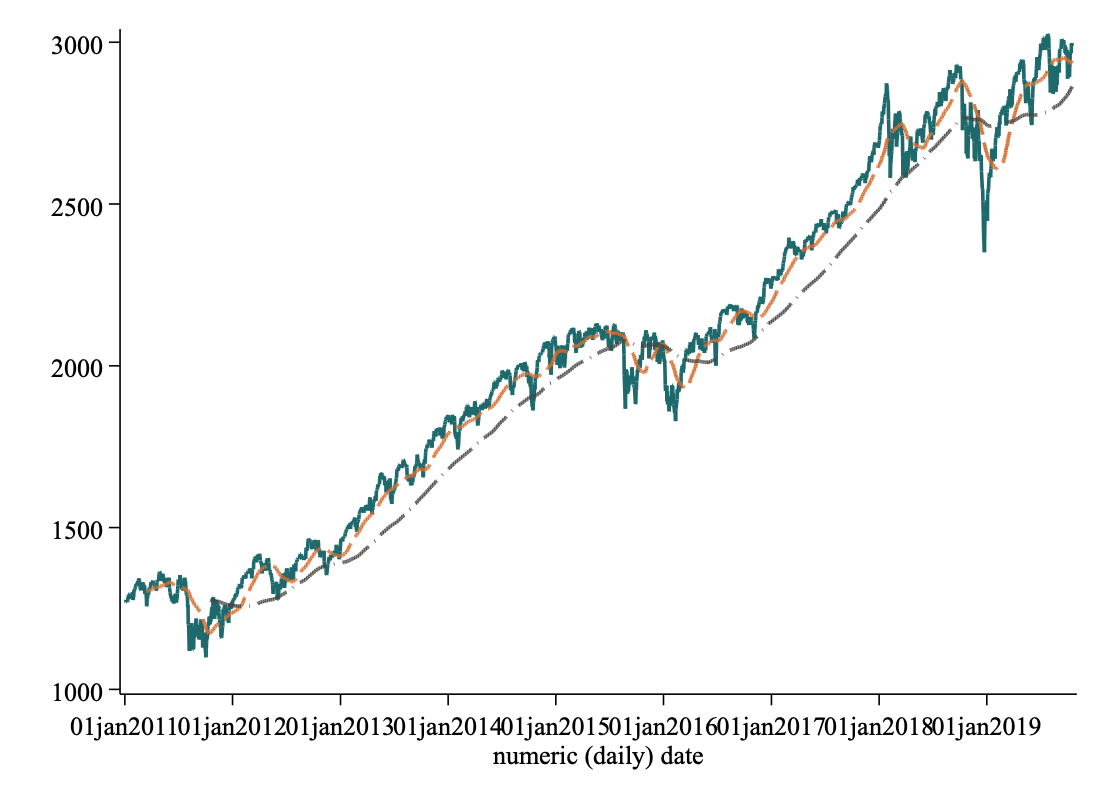## RSI

$$RSI = 100 - \frac{100}{1 + RS}$$

where:

$$RS = \frac{average of upward returns over x days}{average of downward returns over x days}$$## Candle Chart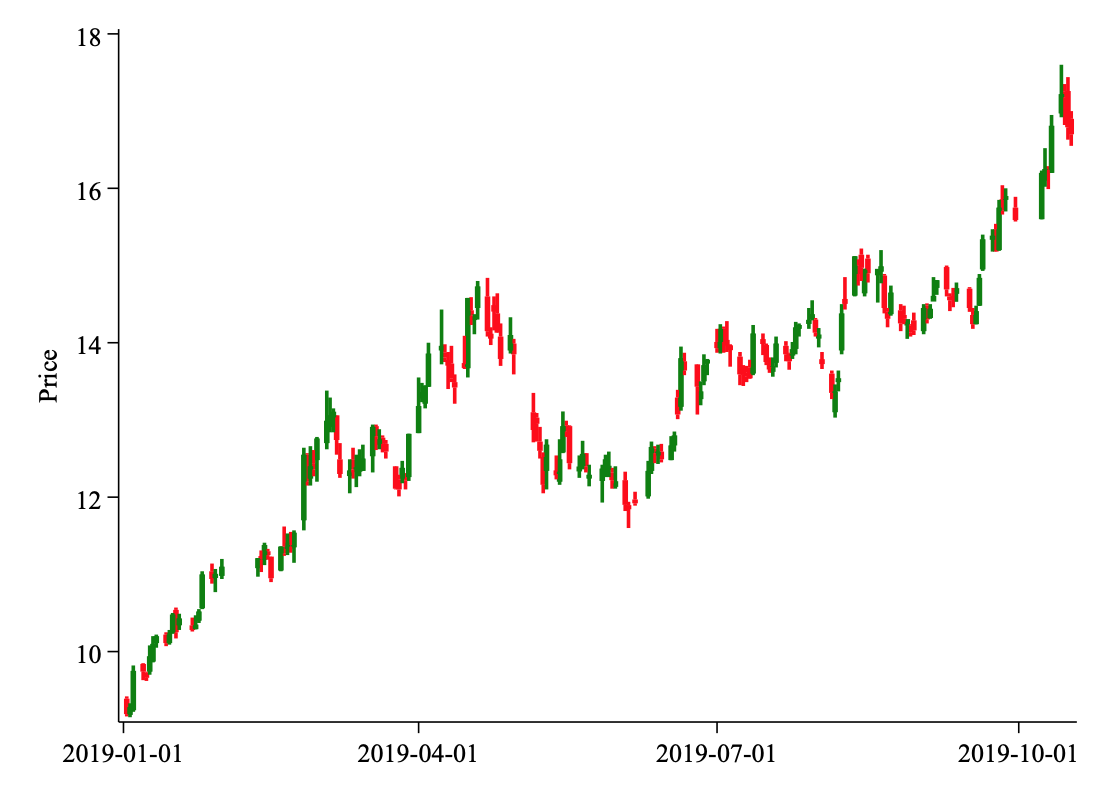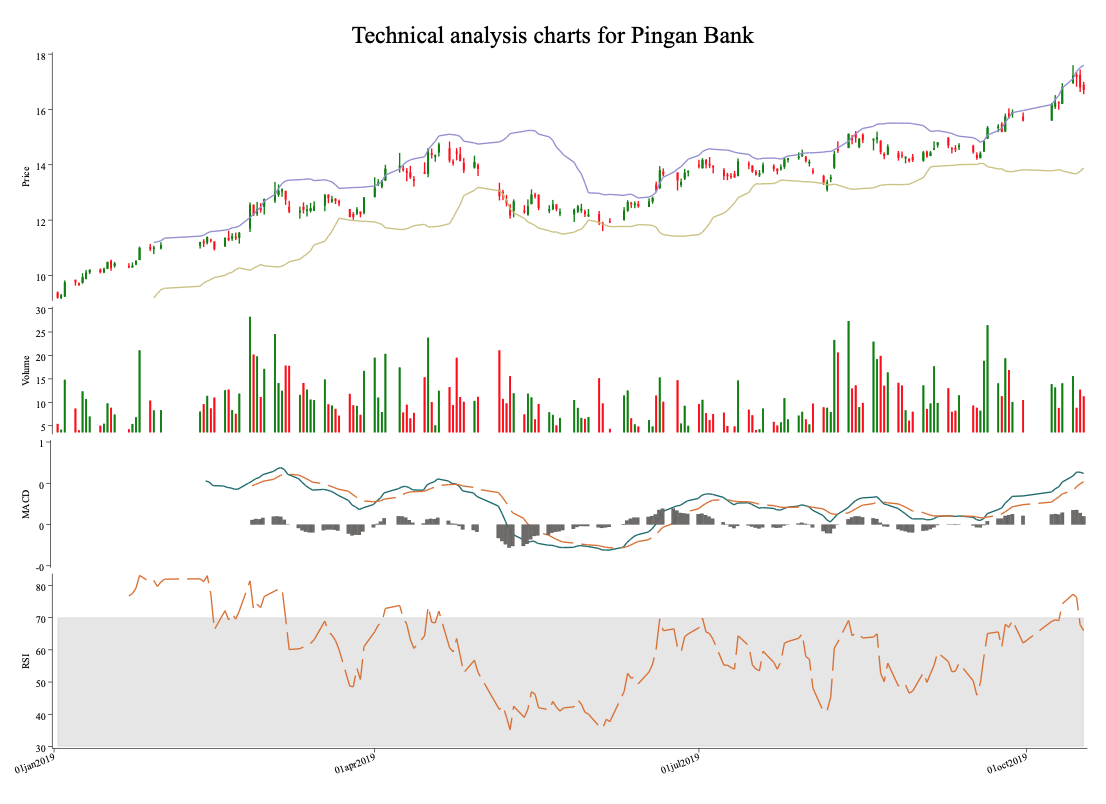## Stata for finance students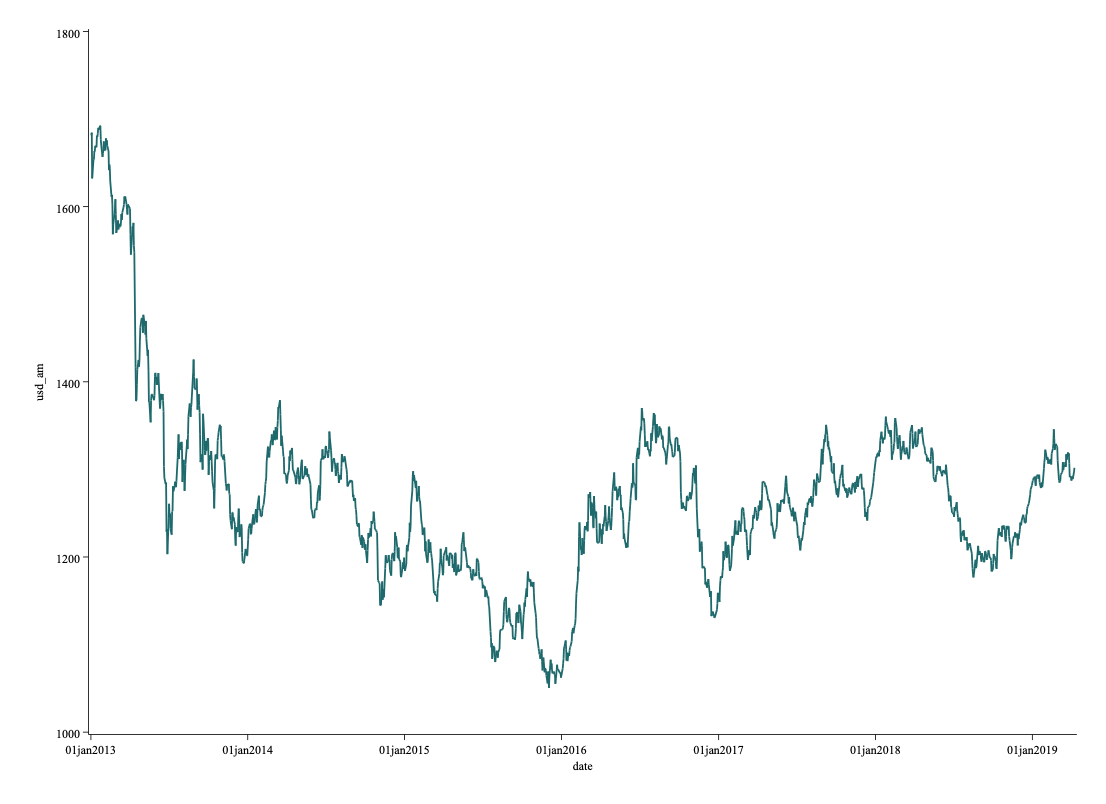## Day-of-the-week effect

Day-of-the-week effect is one of most common return anomalies tested in the literature. It stems from the hypothesis that if securities compensate investors for risk for any holding period then a weekend return should be higher than a daily return. Thus, based on this hypothesis, it would be expected that returns for Fridays to Mondays should be higher than any other mid-week daily return. However, if investors expect higher returns for the weekend they would trade such expectation to take advantage of it. This would eliminate the higher weekend returns. Based on the efficient market hypothesis, such profitable pattern trading should not exist. In fact, if it exists, it would be akin to investors not taking advantage of a profitable trading strategy. Thus, existence of day-of-the-week is considered to be an anomaly: why would investors leave money on the table?

Dicle and Levendis (2014) argue that investors and academic study indexes as well as popular stocks. This leads to less popular stocks to be left not studied. They find no evidence of day-of-the-week effect for indexes. However, based on evaluation of 51 markets in 33 countries, approximately 8% of 37631 stocks present day-of-the-week effect.

The day-of-the-week effect test is quite simple. It basically evaluates returns for each day of the week to be statistically different from each other. For this purposes, a binary variable is assigned to each week day. However, in order to avoid binary variable trap (a.k.a. dummy trap) in the regression, one of the weekdays is left out(usually Wednesday). This allows each week day’s return to compared to the returns on Wednesdays.

It seems no day-of-the-week effect exists. Also it would be best to estimate this model using GARCH(1, 1) model instead of OLS to control for risk.

Still no day-of-the-week effect was founded.

M. B. M.